# Predict Battery State of Charge Using Machine Learning

This example shows how to train a Gaussian process regression (GPR) model to predict the state of charge of a battery in automotive engineering.

Battery state of charge (SOC) is the level of charge of an electric battery relative to its capacity measured as a percentage. SOC is critical information for a vehicle energy management system, and must be accurately estimated to ensure reliable and affordable electrified vehicles (xEV). However, due to the nonlinear temperature, health, and SOC-dependent behavior of Li-ion batteries, SOC estimation is a significant automotive engineering challenge. Traditional approaches to this problem, such as electrochemical models, usually require precise parameters and knowledge of the battery composition, as well as its physical response. In contrast, using a machine learning model is a data-driven approach that requires minimal knowledge of the battery or its nonlinear behavior .

This example trains a Gaussian process regression model to predict the state of charge of a vehicle's Li-ion battery, given time series data that represents various features of the battery such as voltage, current, temperature, and average voltage and current (over the last 500 seconds).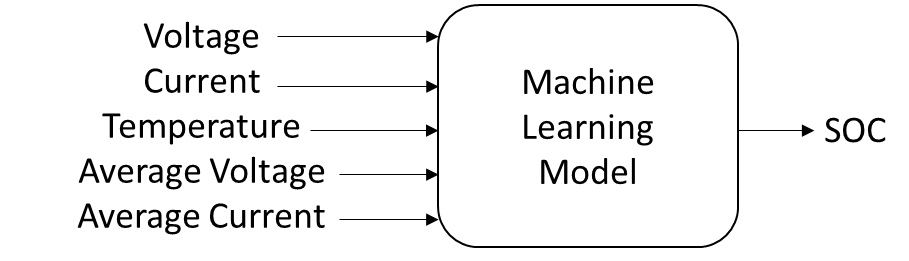After training a regression model, you can use it to create a Simulink® model, and then generate HDL code from the Simulink model for deployment. For details, see Deploy Neural Network Regression Model to FPGA/ASIC Platform.

Load the `batterySOC` data set, which is a subset of the preprocessed data set `LG_HG2_Prepared_Dataset_McMasterUniversity_Jan_2020` from . The optional section Download and Prepare Data shows how to download and prepare the data set to create the subset stored in the `batterySOC.mat` file.

`load("batterySOC.mat")`

The data set contains one table of training data (`trainData`) and four tables of test data (`testDataN10deg`, `testData0deg`, `testData10deg`, and `testData25deg`). The training data is a single sequence of experimental data collected while the battery powered an electric vehicle during driving cycles at four different ambient temperatures, –10, 0, 10, and 25 degrees Celsius. The test data consists of four sequences of experimental data, each collected at one of the same four temperatures. Create a cell array that contains the test data sets.

```testData = cell(4,1); testData{1} = testDataN10deg; testData{2} = testData0deg; testData{3} = testData10deg; testData{4} = testData25deg; testDataName = ["-10^{\circ}C","0^{\circ}C", ... "10^{\circ}C","25^{\circ}C"];```

Each training and test table is a subset of the preprocessed data `LG_HG2_Prepared_Dataset_McMasterUniversity_Jan_2020`, sampled to have a data point every 100 seconds. Preview the first few rows of the training data set.

`head(trainData)`
``` Voltage Current Temperature AverageVoltage AverageCurrent SOC _______ _______ ___________ ______________ ______________ _______ 0.38515 0.75102 0.3031 0.38515 0.75102 0.20642 0.38546 0.75102 0.45607 0.3853 0.75102 0.20642 0.3855 0.75102 0.62209 0.38537 0.75102 0.20642 0.38565 0.75102 0.77026 0.38544 0.75102 0.20642 0.38643 0.75102 0.84667 0.38564 0.75102 0.20642 0.38778 0.75102 0.85707 0.38599 0.75102 0.20642 0.38915 0.75102 0.85474 0.38666 0.75102 0.20642 0.39024 0.75102 0.87646 0.38746 0.75102 0.20642 ```

Each training and test table contains six variables: `Voltage`, `Current`, `Temperature`, `AverageVoltage`, `AverageTemperature`, and `SOC`. Plot the variable values of the training and test data. Select a variable to plot.

```variableToPlot =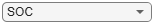"SOC"; figure t = tiledlayout(3,2); nexttile(1,[1,2]) trainDataT = (0:(size(trainData,1)-1))'*100; plot(trainDataT,trainData.(variableToPlot)) title("Training Data") for i = 1 : 4 nexttile currentTestData = testData{i}; currentTestDataT = (0:(size(currentTestData,1)-1))'*100; plot(currentTestDataT,currentTestData.(variableToPlot)) title(join(["Test Data at",testDataName(i)])) end linkaxes(t.Children,"y") xlabel(t,"Time (seconds)") ylabel(t,variableToPlot)```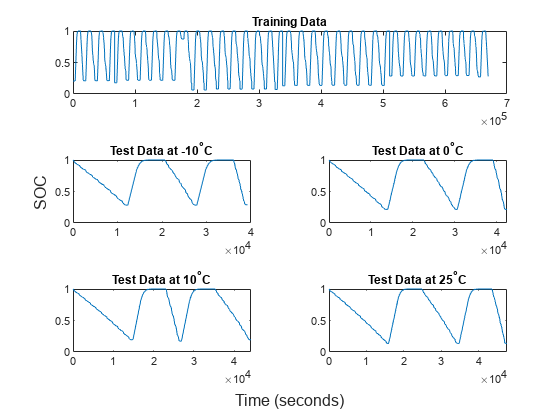All six variables are in the range `[0,1]`. The SOC value for a fully charged battery is 1, and the SOC value for a fully discharged battery is 0. Therefore, the SOC value must be in the range `[0,1]`.

### Train Gaussian Process Regression Model

Train a GPR model by using the `fitrgp` function. Specify the `OptimizeHyperparameters` name-value argument as `["BasisFunction","KernelFunction","Standardize"]`. The function fits a model after finding optimal values for the specified hyperparameters. For reproducibility, set the random seed and use the `expected-improvement-plus` acquisition function. Also, specify `UseParallel=true` to run Bayesian optimization in parallel. This option requires Parallel Computing Toolbox™.

```rng("default") Mdl = fitrgp(trainData,"SOC", ... OptimizeHyperparameters=["BasisFunction","KernelFunction","Standardize"], ... HyperparameterOptimizationOptions= ... struct(AcquisitionFunctionName="expected-improvement-plus",UseParallel=true))```
```Starting parallel pool (parpool) using the 'Processes' profile ... Connected to the parallel pool (number of workers: 6). Copying objective function to workers... Done copying objective function to workers. |==============================================================================================================================| | Iter | Active | Eval | Objective: | Objective | BestSoFar | BestSoFar | BasisFunction| KernelFuncti-| Standardize | | | workers | result | log(1+loss) | runtime | (observed) | (estim.) | | on | | |==============================================================================================================================| | 1 | 6 | Best | 0.00051935 | 98.995 | 0.00051935 | 0.00051935 | constant | matern52 | false | | 2 | 6 | Accept | 0.00054243 | 112.15 | 0.00051935 | 0.00052044 | pureQuadrati | matern52 | false | | 3 | 6 | Accept | 0.00052242 | 98.911 | 0.00051935 | 0.00052111 | constant | matern52 | false | | 4 | 6 | Accept | 0.00052343 | 127.13 | 0.00051935 | 0.0005211 | pureQuadrati | exponential | true | | 5 | 6 | Accept | 0.00058425 | 249.88 | 0.00051935 | 0.00052097 | none | rationalquad | true | | 6 | 6 | Best | 0.00050934 | 105.61 | 0.00050934 | 0.00050952 | constant | matern32 | false | | 7 | 6 | Accept | 0.00052931 | 339.37 | 0.00050934 | 0.00050954 | constant | ardmatern32 | true | | 8 | 6 | Accept | 0.00053765 | 94.526 | 0.00050934 | 0.00050958 | none | matern52 | false | | 9 | 6 | Accept | 0.00061936 | 355.38 | 0.00050934 | 0.00050948 | constant | ardsquaredex | true | | 10 | 6 | Accept | 0.00057228 | 382.03 | 0.00050934 | 0.0005095 | none | ardmatern32 | false | | 11 | 6 | Accept | 0.00053971 | 99.533 | 0.00050934 | 0.00050951 | constant | matern32 | true | | 12 | 6 | Accept | 0.00051496 | 91.813 | 0.00050934 | 0.0005095 | none | matern32 | false | | 13 | 6 | Accept | 0.00057599 | 97.529 | 0.00050934 | 0.00050951 | constant | matern52 | true | | 14 | 6 | Best | 0.00040178 | 109.22 | 0.00040178 | 0.00040204 | constant | exponential | false | | 15 | 6 | Accept | 0.00051895 | 226.34 | 0.00040178 | 0.00040206 | constant | rationalquad | false | | 16 | 6 | Accept | 0.00054575 | 105.36 | 0.00040178 | 0.00040208 | pureQuadrati | matern32 | true | | 17 | 6 | Accept | 0.00053792 | 88.646 | 0.00040178 | 0.0004021 | none | matern32 | true | | 18 | 6 | Accept | 0.00041535 | 115.14 | 0.00040178 | 0.000402 | pureQuadrati | exponential | false | | 19 | 6 | Best | 0.00039856 | 94.383 | 0.00039856 | 0.00039873 | none | exponential | false | | 20 | 6 | Accept | 0.00040507 | 106.15 | 0.00039856 | 0.00039874 | constant | exponential | false | |==============================================================================================================================| | Iter | Active | Eval | Objective: | Objective | BestSoFar | BestSoFar | BasisFunction| KernelFuncti-| Standardize | | | workers | result | log(1+loss) | runtime | (observed) | (estim.) | | on | | |==============================================================================================================================| | 21 | 6 | Accept | 0.00040581 | 102.97 | 0.00039856 | 0.00039873 | constant | exponential | false | | 22 | 6 | Accept | 0.00040278 | 108.56 | 0.00039856 | 0.0003987 | constant | exponential | false | | 23 | 6 | Accept | 0.00040521 | 93.324 | 0.00039856 | 0.00040202 | none | exponential | false | | 24 | 6 | Accept | 0.00040458 | 88.379 | 0.00039856 | 0.00040287 | none | exponential | false | | 25 | 6 | Accept | 0.00040195 | 88.728 | 0.00039856 | 0.00040263 | none | exponential | false | | 26 | 6 | Accept | 0.00041476 | 99.863 | 0.00039856 | 0.00040262 | linear | exponential | false | | 27 | 6 | Accept | 0.00042907 | 96.641 | 0.00039856 | 0.00040262 | none | exponential | true | | 28 | 6 | Accept | 0.00052144 | 100.62 | 0.00039856 | 0.00040262 | pureQuadrati | matern32 | false | | 29 | 6 | Accept | 0.00062455 | 293.96 | 0.00039856 | 0.00040262 | constant | ardsquaredex | false | | 30 | 6 | Accept | 0.00044598 | 98.27 | 0.00039856 | 0.00040262 | linear | exponential | true | ```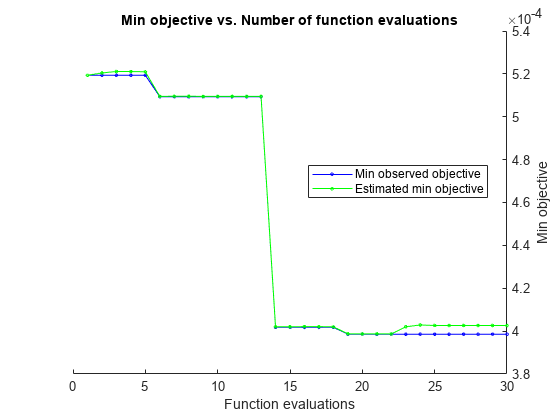```__________________________________________________________ Optimization completed. MaxObjectiveEvaluations of 30 reached. Total function evaluations: 30 Total elapsed time: 848.1224 seconds Total objective function evaluation time: 4269.4267 Best observed feasible point: BasisFunction KernelFunction Standardize _____________ ______________ ___________ none exponential false Observed objective function value = 0.00039856 Estimated objective function value = 0.00040262 Function evaluation time = 94.3834 Best estimated feasible point (according to models): BasisFunction KernelFunction Standardize _____________ ______________ ___________ none exponential false Estimated objective function value = 0.00040262 Estimated function evaluation time = 91.1934 ```
```Mdl = RegressionGP PredictorNames: {'Voltage' 'Current' 'Temperature' 'AverageVoltage' 'AverageCurrent'} ResponseName: 'SOC' CategoricalPredictors: [] ResponseTransform: 'none' NumObservations: 6702 HyperparameterOptimizationResults: [1×1 BayesianOptimization] KernelFunction: 'Exponential' KernelInformation: [1×1 struct] BasisFunction: 'None' Beta: [0×1 double] Sigma: 0.0176 PredictorLocation: [] PredictorScale: [] Alpha: [6702×1 double] ActiveSetVectors: [6702×5 double] PredictMethod: 'Exact' ActiveSetSize: 2000 FitMethod: 'SD' ActiveSetMethod: 'Random' IsActiveSetVector: [6702×1 logical] LogLikelihood: 4.5248e+03 ActiveSetHistory: [1×1 struct] BCDInformation: [] Properties, Methods ```

`Mdl` is a `RegressionGP` model object. You can use the `predict` and `loss` functions of `RegressionGP` to compute the predicted SOC values and mean squared error (MSE), respectively. Recall that the battery SOC values must be in the range `[0,1]`; however, the `predict` function of a GPR model can return values slightly outside of this range. To ensure that values stay in this range, you can apply the `min` and `max` functions to the predicted values. Also, the `loss` function of a GPR model computes the mean squared error (MSE) using the predictions returned by the `predict` function. Instead, you can define a custom loss function to compute an error using the trimmed predicted values in the range `[0,1]`.

If you use a different type of regression model that supports `ResponseTransform`, you can specify the `ResponseTransform` property of the model as `@(y) min(max(y,0),1)`, and use the `predict` and `loss` functions. `RegressionGP` does not support `ResponseTransform`.

Alternatively, you can transform the SOC values to an unbounded range by using the logit transformation. Then, you can train a regression model using transformed data, and transform the predicted value back to the bounded range by using the inverse logit transformation.

### Test Regression Model

Plot the predicted and true SOC values for the training and test data sets. Use the `min` and `max` functions to keep the predicted values in the range `[0,1]`.

```figure t = tiledlayout(3,2); nexttile(1,[1,2]) plot(trainDataT,trainData.SOC) hold on plot(trainDataT,min(max(resubPredict(Mdl),0),1)) hold off ylim([0,1]) legend(["True SOC","Predicted SOC"], ... Location="northoutside",Orientation="horizontal") title("Training Data") predictedSOC = cell(4,1); for i = 1 : 4 nexttile currentTestData = testData{i}; currentTestDataT = (0:(size(currentTestData,1)-1))'*100; predictedSOC{i} = min(max(predict(Mdl,currentTestData),0),1); plot(currentTestDataT,currentTestData.SOC) hold on plot(currentTestDataT,predictedSOC{i}) hold off ylim([0,1]) title(join(["Test Data at",testDataName(i)])) end xlabel(t,"Time (seconds)") ylabel(t,"Battery SOC")```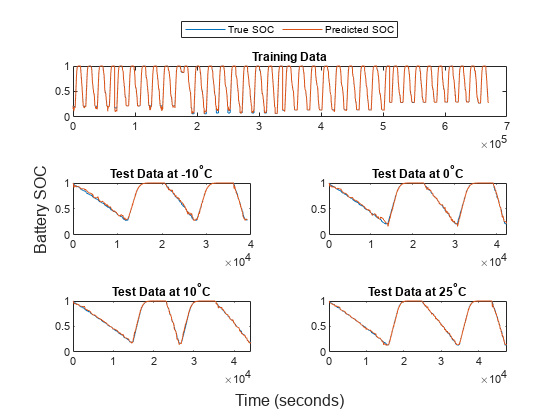Calculate the root mean squared error (RMSE) and maximum absolute error between the predicted SOC and the target SOC for each ambient temperature. Specify custom loss functions to compute the loss values based on the trimmed predicted values.

```testRMSE = NaN(4,1); testMaxAbsError = NaN(4,1); lossFun_RMSE = @(y,ypred,w) sqrt(mean((y-min(max(ypred,0),1)).^2)); lossFun_MaxAbsError = @(y,ypred,w) max(abs(y-min(max(ypred,0),1))); for i = 1: 4 testRMSE(i) = loss(Mdl,testData{i},LossFun=lossFun_RMSE); testMaxAbsError(i) = loss(Mdl,testData{i},LossFun=lossFun_MaxAbsError); end```

Plot the RMSE and maximum absolute error values.

```figure tiledlayout(2,1) nexttile bar(testRMSE) xticklabels(testDataName) xlabel("Ambient Temperature") ylabel("RMSE") nexttile bar(testMaxAbsError) xticklabels(testDataName) xlabel("Ambient Temperature") ylabel("Maximum Absolute Error")```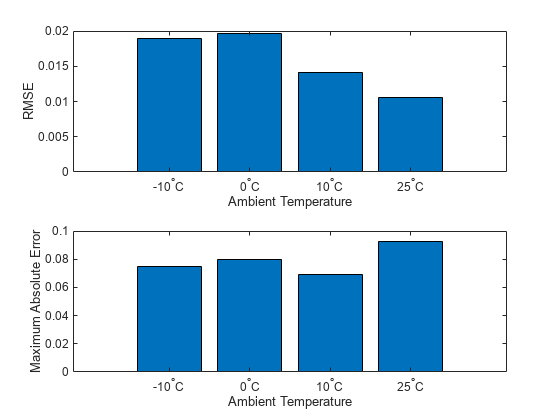Lower values in the RMSE and maximum absolute error plots indicate more accurate predictions for the corresponding temperatures. Larger values in the same plots indicate less accurate predictions for the corresponding temperatures.

As noted earlier, this example uses a subset of the preprocessed data set `LG_HG2_Prepared_Dataset_McMasterUniversity_Jan_2020` from . You can load the subset from the provided file `batterySOC.mat`, or download the data set and preprocess it as described in this section.

Each file in the data set `LG_HG2_Prepared_Dataset_McMasterUniversity_Jan_2020` contains a time series `X` of five predictors (voltage, current, temperature, average voltage, and average current) and a time series `Y` of one target (SOC). Each file represents data collected at a different ambient temperature.

`url = "https://data.mendeley.com/public-files/datasets/cp3473x7xv/files/ad7ac5c9-2b9e-458a-a91f-6f3da449bdfb/file_downloaded";`

Set `downloadFolder` to where you want to download the ZIP file, and set `outputFolder` to where you want to extract the ZIP file.

```downloadFolder = tempdir; outputFolder = fullfile(downloadFolder,"LGHG2@n10C_to_25degC");```

Download and extract the data set `LG_HG2_Prepared_Dataset_McMasterUniversity_Jan_2020`.

```if ~exist(outputFolder,"dir") fprintf("Downloading LGHG2@n10C_to_25degC.zip (56 MB) ... ") filename = fullfile(downloadFolder,"LGHG2@n10C_to_25degC.zip"); websave(filename,url); unzip(filename,outputFolder) end```

Create a file datastore for both the training data and the test data, and specify the read function as the `load` function. The `load` function loads the data from the MAT file into the datastore.

```folderTrain = fullfile(outputFolder,"Train"); folderTest = fullfile(outputFolder,"Test"); fdsTrain = fileDatastore(folderTrain,ReadFcn=@load); fdsTest = fileDatastore(folderTest,ReadFcn=@load);```

Read all data in the datastores.

```trainDataFull = read(fdsTrain); testDataFull = readall(fdsTest); testDataFullN10deg = testDataFull{1}; testDataFull0deg = testDataFull{2}; testDataFull10deg = testDataFull{3}; testDataFull25deg = testDataFull{4};```

Resample the data sets to have one data point every 100 seconds, and compute moving averages by using the helper function `helperMovingAverage`. The code for this function appears at the end of this example.

```idx0 = 1:184257; idx10 = 184258:337973; idx25 = 337974:510530; idxN10 = 510531:669956; trainData0deg = helperMovingAverage(array2table([trainDataFull.X(:,idx0); trainDataFull.Y(idx0)]')); trainData10deg = helperMovingAverage(array2table([trainDataFull.X(:,idx10); trainDataFull.Y(idx10)]')); trainData25deg = helperMovingAverage(array2table([trainDataFull.X(:,idx25); trainDataFull.Y(idx25)]')); trainDataN10deg = helperMovingAverage(array2table([trainDataFull.X(:,idxN10); trainDataFull.Y(idxN10)]')); trainData = [trainData0deg; trainData10deg; trainData25deg; trainDataN10deg]; testDataN10deg = helperMovingAverage(array2table([testDataFullN10deg.X; testDataFullN10deg.Y]')); testData0deg = helperMovingAverage(array2table([testDataFull0deg.X; testDataFull0deg.Y]')); testData10deg = helperMovingAverage(array2table([testDataFull10deg.X; testDataFull10deg.Y]')); testData25deg = helperMovingAverage(array2table([testDataFull25deg.X; testDataFull25deg.Y]'));```

The `batterySOC.mat` file contains the variables `trainData`, `testDataN10deg`, `testData0deg`, `testData10deg`, and `testData25deg`.

### Helper Function

This code creates the `helperMovingAverage` helper function.

```function newTbl = helperMovingAverage(tbl) newTbl = tbl(1:100:end,[1:3,end]); variableNames = ["Voltage","Current","Temperature","SOC"]; newTbl.Properties.VariableNames = variableNames; n = size(newTbl,1); newTbl.AverageVoltage = NaN(n,1); newTbl.AverageCurrent = NaN(n,1); for i = 1 : n newTbl.AverageVoltage(i) = mean(newTbl.Voltage(max(1,i-5):i)); newTbl.AverageCurrent(i) = mean(newTbl.Current(max(1,i-5):i)); end newTbl = movevars(newTbl,"SOC",After="AverageCurrent"); end```

### References

 Kollmeyer, Phillip; Vidal, Carlos; Naguib, Mina; Skells, Michael (2020), “LG 18650HG2 Li-ion Battery Data and Example Deep Neural Network xEV SOC Estimator Script”, Mendeley, Data, V3, https://doi.org/10.17632/CP3473X7XV.3.